# 软件测试 / 测试开发丨 Python 常用数据结构 - 学习笔记

• 2023-05-14
北京
• 本文字数：6418 字

阅读完需：约 21 分钟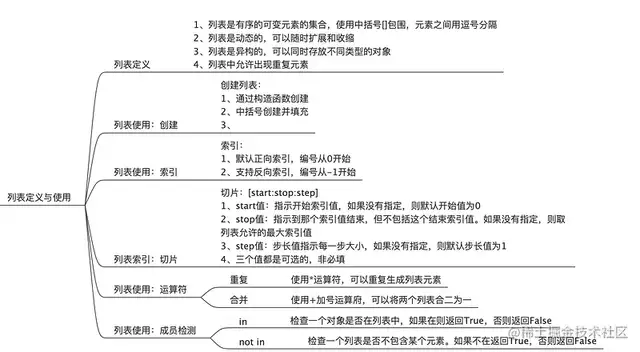#使用中括号包起来的元素就叫做列表var_list = [1,2,3,"a","b","c",True]print(var_list)#前闭后开原则 start：stop：stepprint(var_list[2:-2])print(var_list[2::2])# 1、构建方法 list()li = list()print(type(li), li)li1 = list("hogwards")print(type(li1), li1)# 2、中括号填充元素[]li2 = [1, 2, 3]li3 = ['hello', 'world']li4 = [1, 3.14, 'hogwards', [5, 6, 7]]print(li4)# 列表推导式li5 = [i for i in range(1, 10) if i % 2 == 0]print(type(li5), li5)# 列表使用 索引li6 = [1, 2, 3, 4, 5, 6]# 1、正向索引print(li6)# 2、反向索引print(li6[-3])# 1、重复li_1 =  * 5li_1_1 = ["shirley"] * 5print(li_1)print(li_1_1)# 2、合并li_2 = [1, 4, 7]li_3 = [10, 44, 00]print(li_2 + li_3)# in & not inli_4 = [1, 4, 7, 8]print(1 in li_4)print(10 in li_4)print(1 not in li_4)print(30 not in li_4)

2、列表的常用方法

a、扩展类方法：主要是往列表中添加新的元素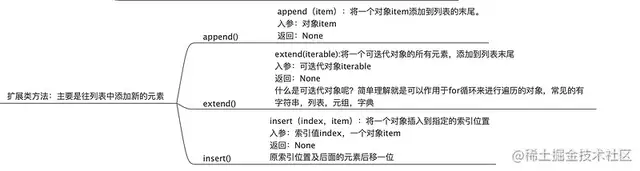# append（）方法li_5 = []print(li_5.append(100))print(len(li_5), li_5)li_5.append('Shirley_Testing')print(len(li_5), li_5)# extend（）方法# 注意extend字符串和列表的区别li_6 = []li_6.extend(['hello', 'world'])li_6.extend('beautiful')print(len(li6), li_6)li_7 = ['shirley', 'test', 'wonderful']li_6.extend(li_7)print(len(li_6), li_6)# insert（）方法li_8 = [1, 2, 3, 4, 5]li_8.insert(3, 100)print(li_8)li_8.insert(2, 'hello wprld')print(li_8)

b.收缩方法：用来移除列表中现有的元素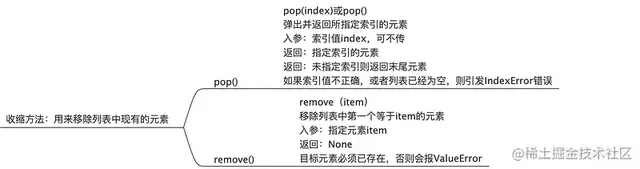# pop()方法li_9 = [5, 34, 22, 6, 7, 9, 65]test_pop = li_9.pop()print(test_pop)print(li_9)print(li_9.pop(3))print(li_9)# IndexError：pop index out of range# 对空列表执行pop方法# 索引超出范围# remove()方法li_10 = ['Effy', 'is', 'is', 'a', 'very', 'very', 'beautiful', 'girl']li_10.remove('is')print(li_10)li_10.remove('very')print(li_10)# 如果元素不存在，去使用remove方法，则会报一个值错误的异常# ValueError: list.remove(x): x not in list# li_10.remove('wonderful')# print(li_10)

c.排序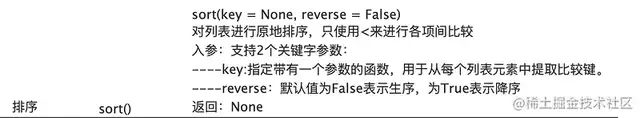# sort()方法li_11 = [1, 4, 6, 8, 9, 0, 2]li_11.sort()print(li_11)li_11.sort(reverse=True)print(li_11)li_12 = ['Effy', '9887676', 'wonderful', 'awesome', '2']li_12.sort(key=len)print(li_12)# 如果列表中有字符串又有数字，则会报错# TypeError: object of type 'int' has no len()

d.反转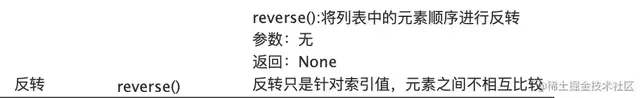# reverse（）方法li_13 = [4,2,7,5,8,0,11]li_13.reverse()print(li_13)# [11, 0, 8, 5, 7, 2, 4]

e.列表嵌套:嵌套列表是指在列表里存放列表；列表的常用方法都适用于嵌套列表

scss复制代码# 创建嵌套列表li_14 = [[1,2,3],['hello','world','python']]print(li_14)li_14.append("test")print(li_14)

f.列表推导式：列表推导式指循环创建列表，相当于 for 循环创建列表的简化版

ini复制代码# 列表推导式li_15 = [1,2,3,4,6,7,8,9]data = [i for i in li_15 if i%2==0]print(data)# for循环 普通写法result = []for i in range (1,11):    if i % 2 == 0:        result.append(i**2)        print(result)# 列表推导式写法data = [i**2 for i in range(1,11) if i % 2 == 0]print(data)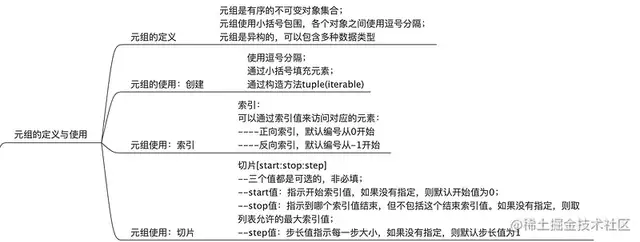#1、直接使用逗号分隔tup1 = 1,2,3,4,5print(type(tup1),tup1)# 2、通过小括号填充元素tup2 = (6,7,8,9,10)print(type(tup2),tup2)# 3、通过构造函数tuple()tup3 = tuple()print(type(tup3),tup3)tup4 = tuple("effy")print(type(tup4),tup4)tup5 = tuple([1,123])print(type(tup5),tup5)# 只有一个元素时，末尾需要加一个逗号tup6 = 1,print(type(tup6),tup6)tup7 = tuple()print(type(tup7),tup7)# 不加逗号，会是一个int类型tup8 = (10)print(type(tup8),tup8)# 元组索引tup9 = tuple("beautiful")# 1、正向索引print(tup9)# 1、反向索引print(tup9[-1])# 切片的使用tup10 = tuple("frustrated")print(tup10)print(tup10[0:3:1])print(tup10[:])print(tup10[:-2])print(tup10[2:5:2])# 特殊的切片写法：逆序print(tup10[::-1])

2、元组常用方法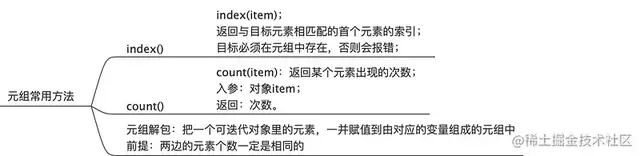# index方法tup11 = tuple("frustrated")print(tup11.index('t'))# 输入元组中不存在的数据# ValueError: tuple.index(x): x not in tuple# print(tup11.index('X'))# count()方法tup12 = tuple("nicenicewonderful")print(tup12.count("n"))print(tup12.count("d"))print(tup12.count("2"))print(tup12.count("c"))

3、解包

# 元组解包tup13 = 1,2,3# 1、传统逐个赋值的方式a = tup13b = tup13c = tup13print(a,b,c)print(type(a))# 解包平行赋值a,b,c = (1,2,3)print(a,b,c)print(type(a))

4、元组与列表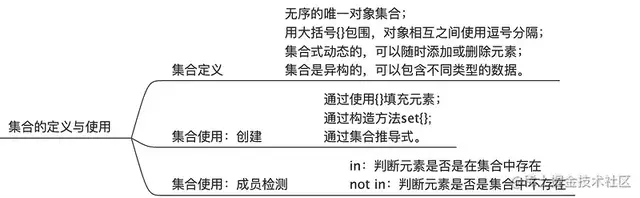# 集合：无序，去重# 1通过使用{}填充元素set_1 = {1,2,3}print(type(set_1),set_1)# <class 'set'> {1, 2, 3}# 2通过构造方法 set{iterable}# 传入字符串set_2 = set("learning python")print(type(set_2),set_2)# <class 'set'> {'p', 'o', 'g', 'r', 'l', ' ', 'y', 't', 'e', 'h', 'i', 'a', 'n'}# 传入列表或者元组set_3 = set([2,5,7,8,7])print(type(set_3),set_3)# <class 'set'> {8, 2, 5, 7}set_4 = set((6,7,8,6))print(type(set_4),set_4)# <class 'set'> {8, 6, 7}# 使用构造方法，不传任何参数时，将会创建一个空的集合对象set_5 = set()print(type(set_5),set_5)# <class 'set'> set()# 3、通过集合推导式# 获取0，1，2，3，4中的偶数部分来构造集合对象set_6 = {i for i in range(5)if i %2 ==0}print(set_6)# {0, 2, 4}# # 获取0，1，2，3，4中的奇数部分的幂方来构造集合对象set_7 = {i**2 for i in range(5) if i % 2 !=0}print(set_7,type(set_7))# {1, 9} <class 'set'># in：判断元素是否是在集合中存在# not in：判断元素是否是集合中不存在set_8 = {"python","234343"}print("python" in set_8)# Trueprint("test" in set_8)# Falseprint("test" not in set_8)# True

2、集合常用方法

a.扩展类方法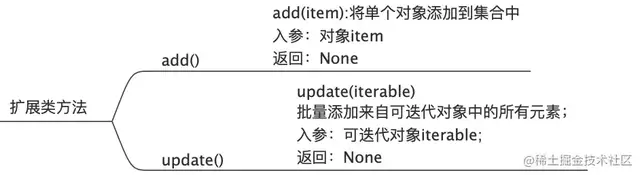# 集合方法add(itme)set_9 = set()set_9.add(1)set_9.add("wonderful")set_9.add(3)print(set_9)# {1, 3, 'wonderful'}# 集合方法update()# 使用update方法传入一个字符串set_9.update("wonderful")print(type(set_9),set_9)# <class 'set'> {1, 3, 'w', 'n', 'e', 'f', 'l', 'o', 'r', 'd', 'wonderful', 'u'}# 使用update方法传入一个列表set_9.update([2,4,6,8])print(set_9,type(set_9))#{'n', 1, 2, 3, 4, 6, 'u', 8, 'wonderful', 'o', 'w', 'r', 'd', 'f', 'l', 'e'} <class 'set'>

b.收缩类方法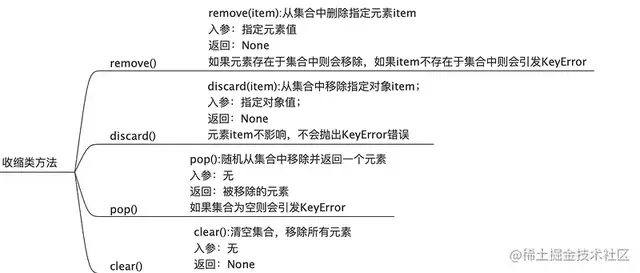# 集合方法remove()set_10 = {1,4,6,'totally'}set_10.remove(6)print(set_10)# {1, 'totally', 4}# remove一个不存在的值，则会报错# set_10.remove(100)# print(set_10)##     set_10.remove(100)# KeyError: 100# 使用remove方法，每次嗨必须确保元素必须在集合中存在，如果嫌麻烦可以使用discard方法# 集合方法discard()set_11 = {46,34,75,"test"}set_11.discard(100)print(set_11,type(set_11))# {34, 75, 46, 'test'} <class 'set'>   即使元素不存在，不会报错set_11.discard(75)print(set_11,type(set_11))# {34, 46, 'test'} <class 'set'>  元素存在，直接移除# 集合方法 pop()set_12 = {6,7,2,"Effy"}print(set_12.pop())# 2print(set_12)# {'Effy', 6, 7}# 集合方法 clear()set_13 = {3,2,7,4,"hello","world"}set_13.clear()print(set_13)# set()

c.集合运算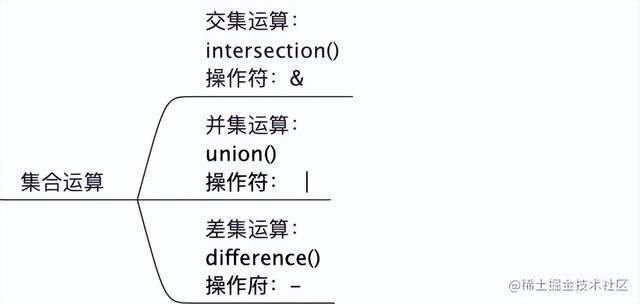# 集合运算# 交集运算  intersection()  操作符 &set_14 = {1,5,7,9}set_15 = {6,2,9,11}data = set_14.intersection(set_15)print(data)# {9}# 也可以使用 & 符号print(set_14 & set_15)# {9}#并集运算  union()   操作符 |set_16 = {1,2,4,6,7}set_17 = {6,9,8}print(set_16.union(set_17))# {1, 2, 4, 6, 7, 8, 9}print(set_16 | set_17)# {1, 2, 4, 6, 7, 8, 9}#差集运算  difference()   操作符 -set_18 = {1,2,3,5,6,7}set_19 = {1,3,5,7,8,9,10}print(set_18.difference(set_19))# {2, 6}print(set_18 - set_19)# {2, 6}print(set_19.difference(set_18))# {8, 9, 10}print(set_19 - set_18)# {8, 9, 10}

d.集合推导式：类似列表推导式，同样集合支持集合推导式；

# 集合推导式print({i for i in range(10) if i%2==0})# {0, 2, 4, 6, 8}# 实例 寻找 beautiful 和wonderful的共同字母# 一般for循环set_20 = set()for i in "beautiful":    if i in "wonderful":        set_20.add(i)print(set_20)# {'l', 'f', 'e', 'u'}# 推导式（使代码非常的简洁）print({i for i in "beautiful" if i in "wonderful"})# {'e', 'l', 'f', 'u'}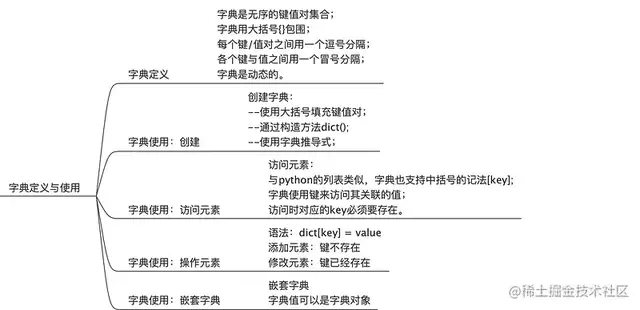"""字典字典的键：通常是一个字符串，关联的值可以是任意的python对象，包括字符串，列表，元组，集合和字典等等；在字典中，每一个唯一键有与之相关联的值；字典可以有多个键值对，与键关联的值可以是任意的对象关于键和值有一定的约束条件：e.g.字典的键可以是任意不可变的类型，通常是字符串和数值，在同一个字典层级内，键必须是唯一的，不能出现重复，字典的值则可以是任意的python对象，比如字符串，列表，元组，集合，还可以是另一个字典对象等等；"""""" 字典使用：创建""""""1、使用大括号填充键值对"""dict_1 = {"name":"Kety perry","age":18}print(type(dict_1),dict_1)# <class 'dict'> {'name': 'Kety perry', 'age': 18}# 如果不赋值，则会得到一个空的字典对象dict_2 = {}print(type(dict_2),dict_2)# <class 'dict'> {}"""2、使用字典的构造方法- 使用dict关键字"""dict_3 = dict()# 传入可迭代的对象，一般是类似于键和值成对出现的数据，如果不填的话，会得到一个空的字典print(type(dict_3),dict_3)# <class 'dict'> {}dict_4 = dict([("name","Taylor Swift"),("age",22)])print(dict_4,type(dict_4))# {'name': 'Taylor Swift', 'age': 22} <class 'dict'>"""3、字典推导式"""dict_5 = {i:j for i,j in [("name","Taylor Swift"),("age",22)]}print(dict_5,type(dict_5))# {'name': 'Taylor Swift', 'age': 22} <class 'dict'>"""字典使用：访问元素"""dict_6 = {'name': 'Taylor Swift', 'age': '22'}# 访问存在的keyprint(dict_6['name'])# Taylor Swiftprint(dict_6["age"])# 22# 访问不存在的key# print(dict_6["hobby"])# KeyError: 'hobby'"""操作元素"""dict_7 =  {'name': 'Taylor Swift', 'age': '22'}# 赋值已存在的keydict_7["name"] = "Adele"print(dict_7)# {'name': 'Adele', 'age': '22'}dict_7["age"] = 27print(dict_7)# {'name': 'Adele', 'age': 27}# 赋值不存在的key,实现新增一个键值对的操作dict_7["hobby"] = "dancing"print(dict_7)# {'name': 'Adele', 'age': 27, 'hobby': 'dancing'}"""字典使用 嵌套字典"""# 在我们的测试工作中，经常打交道的接口响应报文，一般都是这种嵌套的复杂的字典结构# 访问元素和操作嵌套字典的元素是一样的道理dict_8 = {"name":"Taylor Swift","class":"六年二班","course":{"English":100,"Chinese":98,"Math":94}}# 获取课程Chinese的分数print(dict_8["course"]["Chinese"])# 98# 将课程数学分数改成100dict_8["course"]["Math"] = 100print(dict_8["course"]["Math"])print(dict_8)# 100# {'name': 'Taylor Swift', 'class': '六年二班', 'course': {'English': 100, 'Chinese': 98, 'Math': 100}}

2、字典常用方法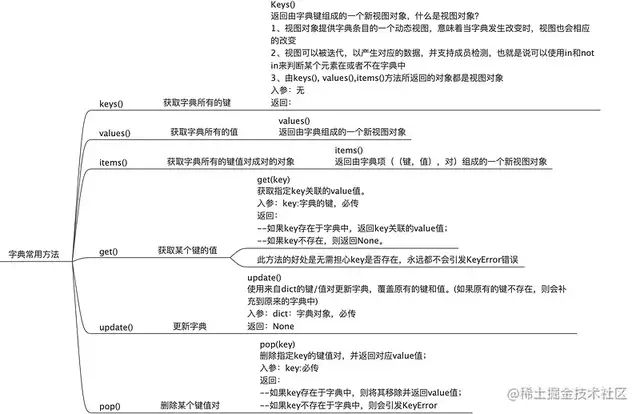"""字典常用方法""""""keys() | values() | items()"""dict_9 = {"name":"Taylor Swift","class":"六年二班","course":"python"}print(dict_9.keys())# dict_keys(['name', 'class', 'course'])print(dict_9.values())# dict_values(['Taylor Swift', '六年二班', 'python'])print(dict_9.items())# dict_items([('name', 'Taylor Swift'), ('class', '六年二班'), ('course', 'python')])# 转化成列表print(list(dict_9.keys()))print(list(dict_9.values()))print(list(dict_9.items()))# ['name', 'class', 'course']# ['Taylor Swift', '六年二班', 'python']# [('name', 'Taylor Swift'), ('class', '六年二班'), ('course', 'python')]"""get(key)"""dict_10 = {"name":"Taylor Swift","class":"六年二班","course":"python"}# 1、访问存在的keyprint(dict_10.get("name"))print(dict_10.get("course"))# Taylor Swift# python# 2、访问不存在的keyprint(dict_10.get("hobby"))# None"""update()"""dict_11 = {"name":"Taylor Swift","class":"六年二班","course":"python"}dict_12 = {"age":20,"course":"Java"}dict_11.update(dict_12)print(dict_11)# {'name': 'Taylor Swift', 'class': '六年二班', 'course': 'Java', 'age': 20}"""pop()"""dict_13 =  {'name': 'Taylor Swift', 'class': '六年二班', 'course': 'Java', 'age': 20}# 1、删除已经存在的Keydict_14 = dict_13.pop("course")print(dict_13)# {'name': 'Taylor Swift', 'class': '六年二班', 'age': 20}print(dict_14)# Java# 2、删除不存在的key,会抛错# dict_13.pop("hobby")# print(dict_13)# KeyError: 'hobby'

3、字典推导式：字典推导式：可以从任何以键值对作为元素的可迭代对象中构建出字典。

"""实例：给定一个字典对象{'a':1,'b':2,'c':3}，找出其中所有大于1 的键值对，同时value进行平方运算"""dict_15 = {'a':1,'b':2,'c':3}dict_16 = {k:v**2 for k,v in dict_15.items() if v>1}print(dict_16)# {'b': 4, 'c': 9}# 普通for循环dict_17 = {'a':1,'b':2,'c':3}dict_18 = {}for k,v in dict_17.items():    if v > 1:        dict_18[k]=v**2print(dict_18)# {'b': 4, 'c': 9}"""实例：给定一个字典对象，请使用字典推导式，将它的key和value分别进行交换，也就是key变成值，值变成key输入：{'a':1,'b':2,'c':3}输出：{1: 'a', 2: 'b', 3: 'c'}"""dict_19 = {'a':1,'b':2,'c':3}dict_20 = {v:k for k,v in dict_19.items()}print(dict_19)print(dict_20)# {1: 'a', 2: 'b', 3: 'c'}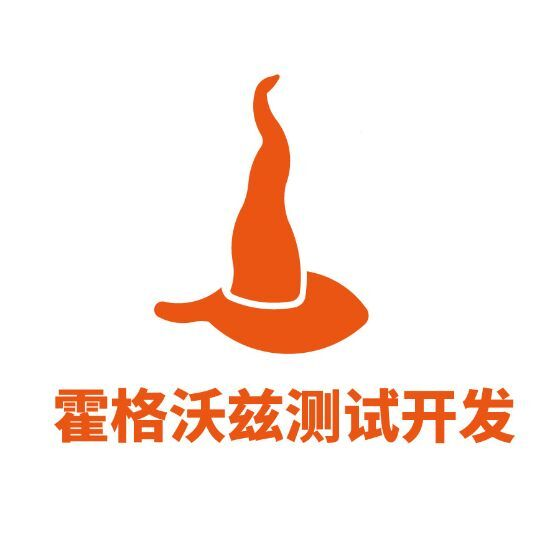## 评论